# 实现边坍塌的网格简化方法

一、算法介绍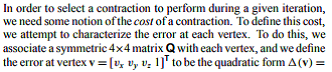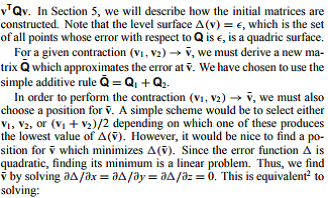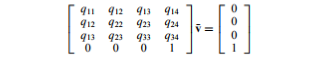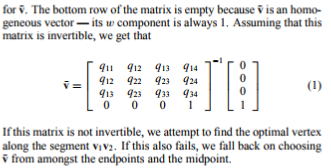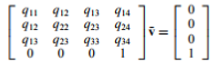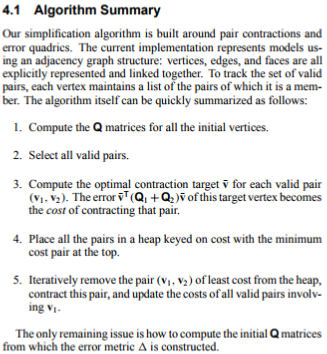1.对所有的初始顶点计算Q矩阵.

2.选择所有有效的边（这里取的是联通的边，也可以将距离小于一个阈值的边归为有效边）

3.对每一条有效边(v1,v2)，计算最优抽取目标v¯.误差v¯T(Q1+Q2)v¯是抽取这条边的代价（cost）

4.将所有的边按照cost的权值放到一个堆里

5.每次移除代价（cost）最小的边，并且更新包含着v1的所有有效边的代价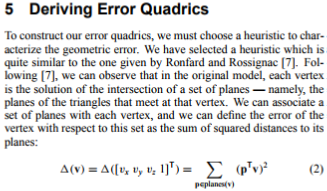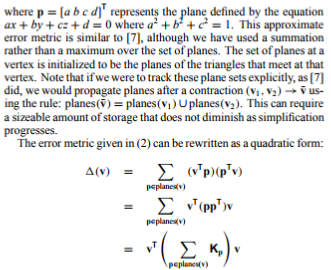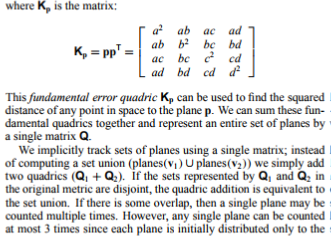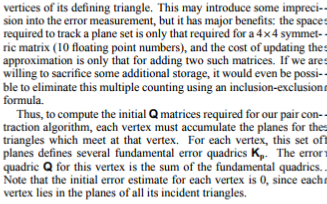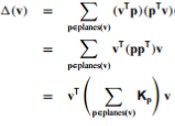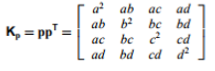``	void setRatio(double);//设置简化率void setLeftFaceNum(int);//设置最终剩余的面片数void input();//读入初始结果void start();//开始简化void output();//输出结果//点的相关操作Matrix calVertexDelta(int);MyVec3d calVertexPos(Edge&,Matrix);//根据一个边两个点的误差矩阵计算收缩后的点位置//边的相关操作void calVAndDeltaV(Edge&);//计算并确定一个边v和deltaV;	``

``````MyVec3d MeshSimplify::calVertexPos(Edge& e,Matrix m){MyVec3d mid = (vGroup->group[e.v1].pos + vGroup->group[e.v2].pos) / 2;  //中点，应对退化情况m.mat = 0;m.mat = 0;m.mat = 0;m.mat = 1;Vector4 Y(0,0,0,1);Solve* solve = new Solve(m,Y);Vector4 ans = solve->getAns();if(ans.v > Config::EPS)return MyVec3d(ans.v,ans.v,ans.v);elsereturn mid;
}``````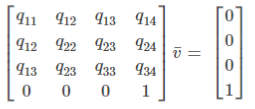``````Matrix MeshSimplify::calVertexDelta(int _id){Matrix ans;Vertex* p = &(vGroup->group[_id]);for(set<int>::iterator it1 = p->connectVertexes.begin();it1 != p->connectVertexes.end();it1++){for(set<int>::iterator it2 = p->connectVertexes.begin();it2 != p->connectVertexes.end();it2++){if((*it1) < (*it2) && (vGroup->group[(*it1)].isExistConnectVertex(*it2))){Vertex* v1 = &(vGroup->group[(*it1)]);Vertex* v2 = &(vGroup->group[(*it2)]);MyVec3d n = ( (v1->pos) - (p->pos) ).getCross( (v2->pos) - (p->pos)).getUnitVectorOfThis();Vector4 tmp(n.x, n.y, n.z, -(p->pos.getDot(n)));for(int i = 0;i < 4;i++){for(int j = 0;j < 4;j++){ans.mat[i][j] += tmp.v[i] * tmp.v[j];}}}}}return ans;
}``````

``````void MeshSimplify::calVAndDeltaV(Edge& e){Matrix mat = calVertexDelta(e.v1) + calVertexDelta(e.v2);//计算完顶点的误差然后求和e.v = calVertexPos(e,mat);//确定一个点收缩后的点	Vector4 X(e.v.x, e.v.y, e.v.z, 1.0);if (vGroup->getCommonVertexNum(e.v1, e.v2) != 2) {e.deltaV = 0;return;}double pri = 0;for (int i = 0; i < 4; i++) {double p = 0;for (int j = 0; j < 4; j++)p += X.v[j] * mat.mat[i][j];pri += p * X.v[i];}e.deltaV = pri;
}``````

``````void MeshSimplify::input(){int cntv=0,cntf=0;char s;int tmp = 0;while (scanf("%s", s) == 1){tmp++;switch (s) {case '#': fgets(s, sizeof s, stdin); break;case 'v': {cntv++;double x, y, z;scanf("%lf %lf %lf", &x, &y, &z);vGroup -> addVertex(Vertex(x,y,z));break;}case 'f': {cntf++;cntFace++;int a, b, c;scanf("%d%d%d", &a, &b, &c);//建立邻接关系,已检查vGroup->group[a].addConnectVertex(b);vGroup->group[a].addConnectVertex(c);vGroup->group[b].addConnectVertex(a);vGroup->group[b].addConnectVertex(c);vGroup->group[c].addConnectVertex(a);vGroup->group[c].addConnectVertex(b);break;}default: break;}}//下面将边加入到边堆中for(int i = 1;i <= vGroup->cntVertex;i++){//遍历所有顶点for(set<int>::iterator it = vGroup->group[i].connectVertexes.begin();//遍历某个顶点的所有邻接点it != vGroup->group[i].connectVertexes.end();it++){if(i < (*it))break;Edge e((*it),i);calVAndDeltaV(e);//每创建一条边就要计算缩后点和误差eHeap->addEdge(e);//将一条边加入其中}}cntDelFace = (int)((1-ratio) * cntFace);//计算应该剩下多少个面
}``````

``void MeshSimplify::start(){for(int i = 0;i < cntDelFace;i += 2){//开始删边	Edge e = eHeap->getMinDelta();Vertex* v1 = &(vGroup->group[e.v1]);Vertex* v2 = &(vGroup->group[e.v2]);Vertex v0 = e.v;int v0_id = vGroup->addVertex(v0);Vertex* pV0 = &(vGroup->group[v0_id]);//定位到缩后的点set<int> connectV;//pV0的邻接点connectV.clear();eHeap->delEdge(e);//打上边已经删除的标记for(set<int>::iterator it = v1->connectVertexes.begin(); it != v1->connectVertexes.end();it++){if((*it)!=v2->id){eHeap->delEdge( Edge((*it),v1->id));vGroup->group[(*it)].delConnectVertex(v1->id);connectV.insert((*it));}}for(set<int>::iterator it = v2->connectVertexes.begin(); it != v2->connectVertexes.end();it++){if((*it)!=v1->id){eHeap->delEdge( Edge((*it),v2->id));vGroup->group[(*it)].delConnectVertex(v2->id);connectV.insert((*it));}}//将原来u，v的结点的邻接点集合中加入新点ofor (set<int>::iterator it = connectV.begin();it != connectV.end(); it++) {vGroup->group[(*it)].addConnectVertex(v0_id);vGroup->group[v0_id].addConnectVertex(*it);}vGroup->isDeleted[v1->id] = true;//标记结点已经被删除vGroup->isDeleted[v2->id] = true;//给新点加边for (set<int>::iterator it = connectV.begin(); it != connectV.end(); it++) {Edge e((*it),v0_id);calVAndDeltaV(e);eHeap->addEdge(e);}}}``

Start的一开始就是根据现在堆里的信息选择cost最小的边进行删除，对应边置上删除的标记并且得到了我们需要的收缩点的，根据新的点的信息结合原来边的点的邻接点的信息来组成新的边，更新这个信息，最后最重要的，不要忘了根据新的信息维护你的堆，以便下一次迭代使用。

``````int main(int argc, char* argv[])
{string inputname="";string outputname="";string outputsurfacenumber="";//cout<<"输入原obj文件名，结果obj文件名，简化率"<<endl;cin>>inputname>>outputname>>outputsurfacenumber;const char* inputfile=inputname.c_str();const char* outputfile=outputname.c_str();const char* simply_rate=outputsurfacenumber.c_str();MeshSimplify* meshSimplify = new MeshSimplify();freopen(inputfile,"r",stdin);freopen(outputfile,"w",stdout);meshSimplify->setRatio(atof(simply_rate));//设置简化率meshSimplify->input();//读入meshSimplify->start();//开始简化meshSimplify->output();//输出freopen("CON", "r", stdin);freopen("CON", "r", stdout);objModel.load(outputname);glutInit(&argc, argv);init();glutDisplayFunc(display);glutReshapeFunc(reshape);glutMouseFunc(moseMove);glutMotionFunc(changeViewPoint);glutIdleFunc(myIdle);glutMainLoop();return 0;
}``````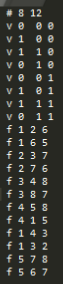Opengl显示为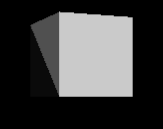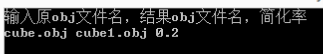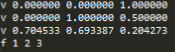Opengl显示为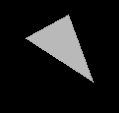（代码参考：https://github.com/lihy96/MeshSimplify）